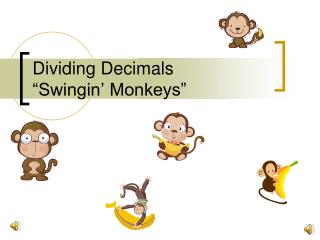DownloadDownload PresentationDividing Decimals “Swingin’ Monkeys”

# Dividing Decimals “Swingin’ Monkeys”

Télécharger la présentation## Dividing Decimals “Swingin’ Monkeys”

- - - - - - - - - - - - - - - - - - - - - - - - - - - E N D - - - - - - - - - - - - - - - - - - - - - - - - - - -
##### Presentation Transcript

1. Dividing Decimals“Swingin’ Monkeys”

2. Swinging Monkeys! Follow these steps and you will be great at dividing decimals! Remember: Whatever the OUTSIDE monkeys do – the INSIDE monkeys do also! Monkey See; Monkey Do!

3. Division Vocabulary “Roof” Quotient “Outside” Divisor “Inside” Dividend

4. Division Vocabulary 12.64 ÷ .2 12.64 divided by .2

5. Steps in Dividing Decimals • Make the divisor(outside number) a whole number by moving (swingin’) the decimal to the right as many places as it takes to make it a WHOLE NUMBER. • In MONKEY TERMS: Whatever the outside monkey does (how far it swings) the inside monkey does also. Then the monkey climbs up and sits on the roof!

6. Swingin’ Monkeys Divisor Moved (swung) the decimal point 1 place to the right Swingin’ Monkey

7. Steps in Dividing Decimals 2. Move (swing) the decimal point in the dividend the same number of places as the divisor. Dividend Moved (swung) the decimal point 1 place to the right

8. Steps in Dividing Decimals – Swingin’ Monkeys

9. Steps in Dividing Decimals – Swingin’ Monkeys 3. Place (swing) the decimal point in the answer (quotient) lined up with the decimal point in the dividend (on the roof).

10. Steps in Dividing Decimals 4. Now, divide as usual!

11. Steps in Dividing Decimals 4. Divide the numbers. Make sure the decimal point stays lined up. 6 3 2 12 6 6 4

12. What is actually happening . . . • When you move the decimal one place to the right to create a WHOLE number – you are actually multiplying it by 10! • When you move the decimal two places to the right to create a WHOLE number – you are actually multiplying it by 100! • Three?

13. Let’s Practice Remember: The more neatly you write and keep the numbers lined up, the BETTER!

14. Your Turn - Practice • 14.2 ÷ 0.2 = • 25.5 ÷ 1.5 =